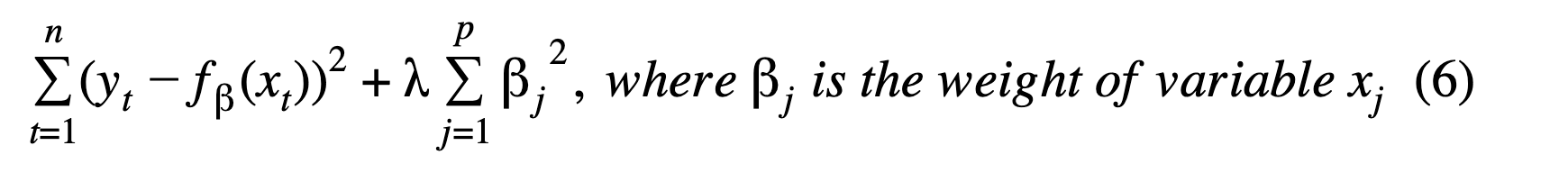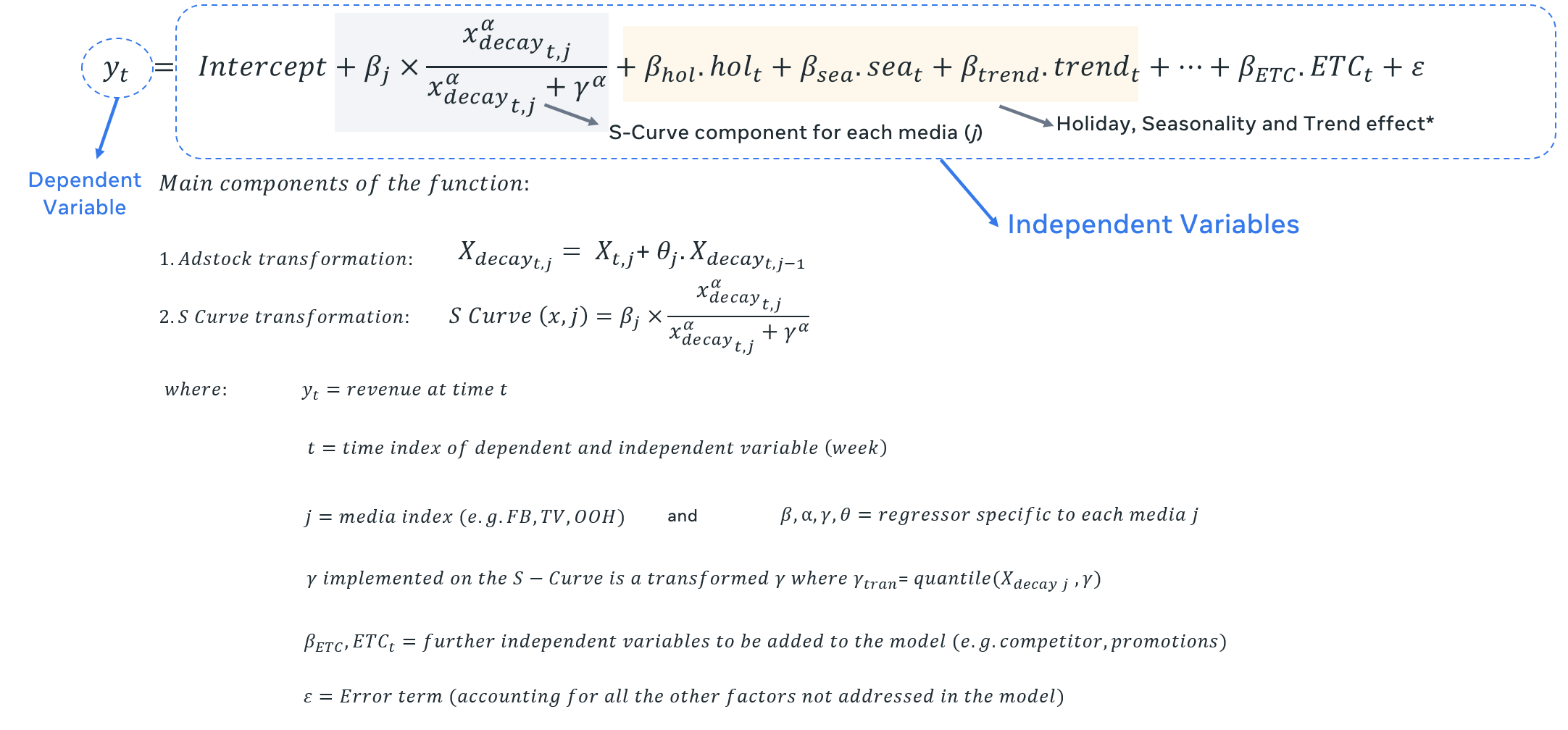# Ridge Regression

In order to address multicollinearity among many regressors and prevent overfitting we apply a regularization technique to reduce variance at the cost of introducing some bias. This approach tends to improve the predictive performance of MMMs. The most common regularization, and the one we are using in this code is Ridge regression. The mathematical notation for Ridge regression is:If we go a bit deeper into the actual components we will be using within the model specification, besides the lambda penalization term above, we can identify the following formula:Below the code where we execute this part, remember you will find it under the ‘func.R’ script:

``        #####################################        #### fit ridge regression with x-validation        cvmod <- cv.glmnet(x_train                           ,y_train                           ,family = "gaussian"                           ,alpha = 0 #0 for ridge regression                           ,lower.limits = lower.limits                           ,upper.limits = upper.limits                           ,type.measure = "mse"        )``